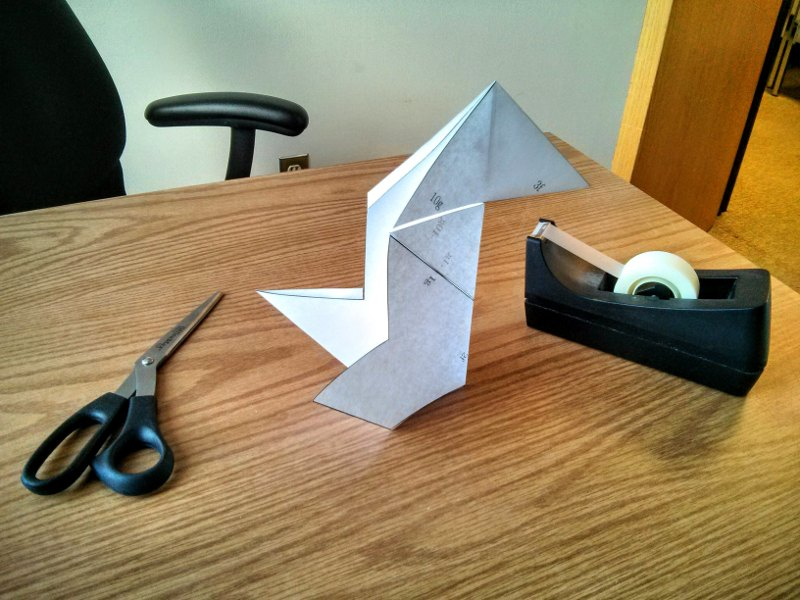# Isospectral drums

## Background

In 1966 Kac asked the question "Can one hear the shape of a drum?", i.e. do the frequencies of a drum's vibration fully determine its shape? For plane domains with fixed boundary (which are the closest to the physical drums), this question was answered in the negative by Gordon, Webb and Wolpert (GWW) in 1992. Their construction based on the so-called Sunada method (1984) that uses representation theory. Various proofs, generalizations and applications of this construction exist; in particular one recent application is in the study of Dirac points in the spectrum of graphene, by A.Comech and myself. This page provides the instructions for proving the isospectrality of Gordon--Webb--Wolpert domains. The proof was constructed by Berard, adapted to folding by Chapman; blueprints were made by myself. All references are given below.

## Instructions

1. Print out the 3 drawings of domain A, number 1, number 2 and number 3. These will represent three copies of the same eigenfunction of the domain.
2. Turning the domain upside down corresponds to multiplying the function (which describes displacement from flat equilibrium) by -1.
3. We will be gluing the copies of the functions along edges marked by a number followed by "g". Negative number means that this copy will be turned over. Convince yourself that such a gluing results in a continuous and continuously differentiable function.
4. We will fold the copies along lines marked by a number followed by "f". Folding corresponds to adding the values of the function (or rather subtracting: note that one of them is multiplied by -1). Convince yourself that the new function takes value zero along the fold.
5. Turn over the copy with the (very long) edge marked by -1g and glue it to the copy with the edge marked by 1g.
6. Fold the glued copies along the line 2f. When folding, make sure the solid line is on the outside of the fold and dashed line is on the inside.
7. Find the line 3f and fold along it. Carry on sequentially.
8. In the end you should get the triple cover of domain 3 with correct boundary conditions.

## Pictures of work in progress

1. After step 1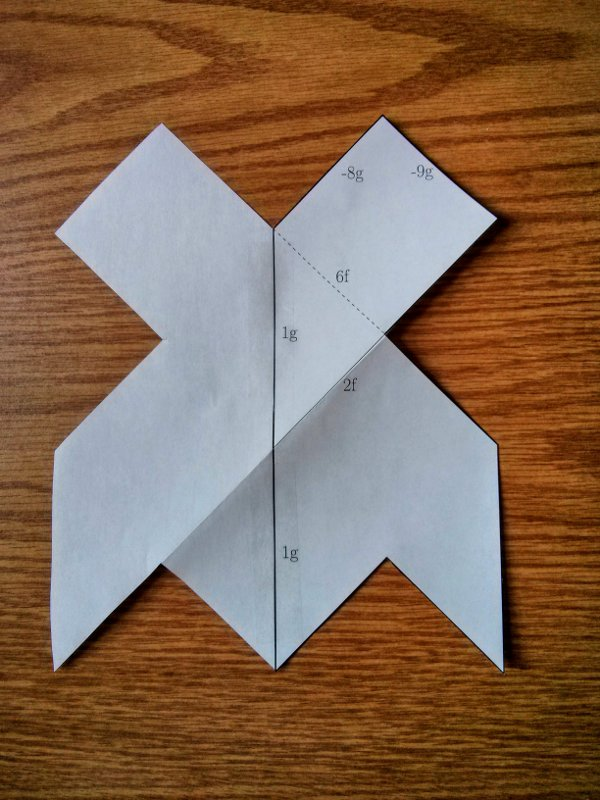2. After step 2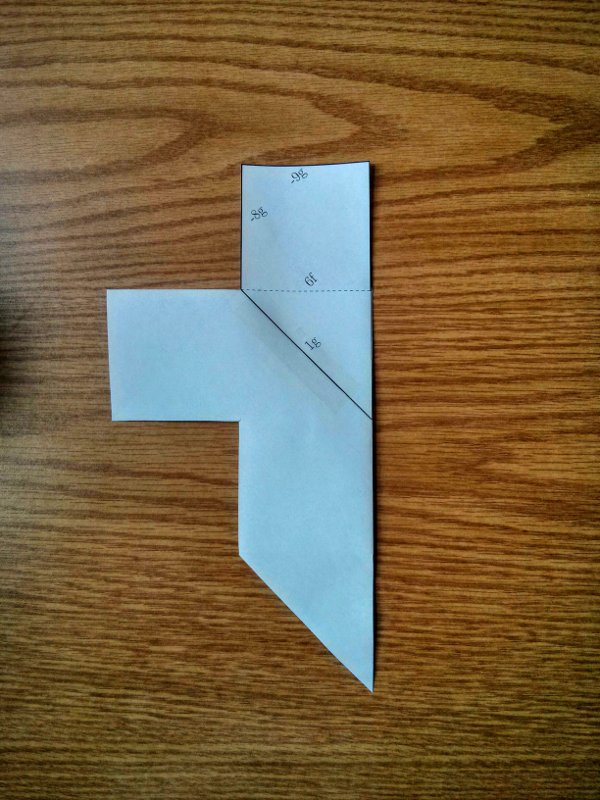3. After steps 3 and 4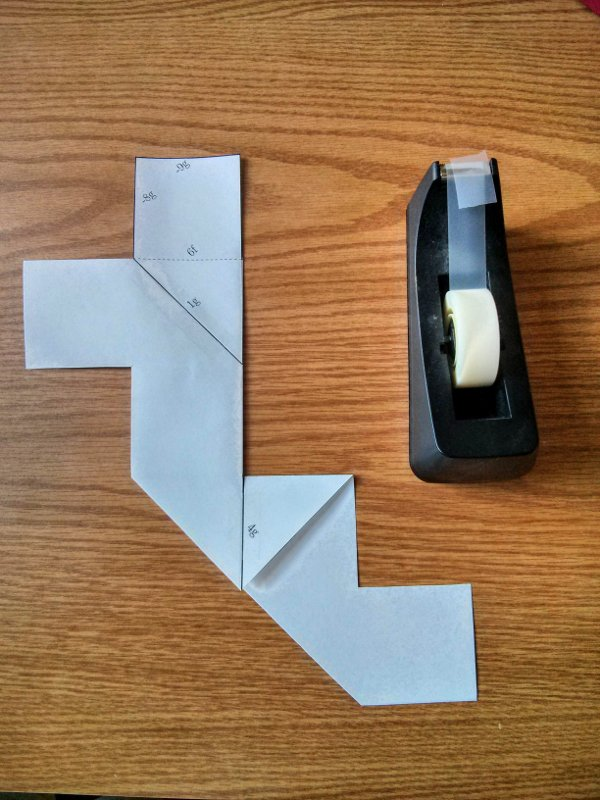4. After steps 5, 6 and 7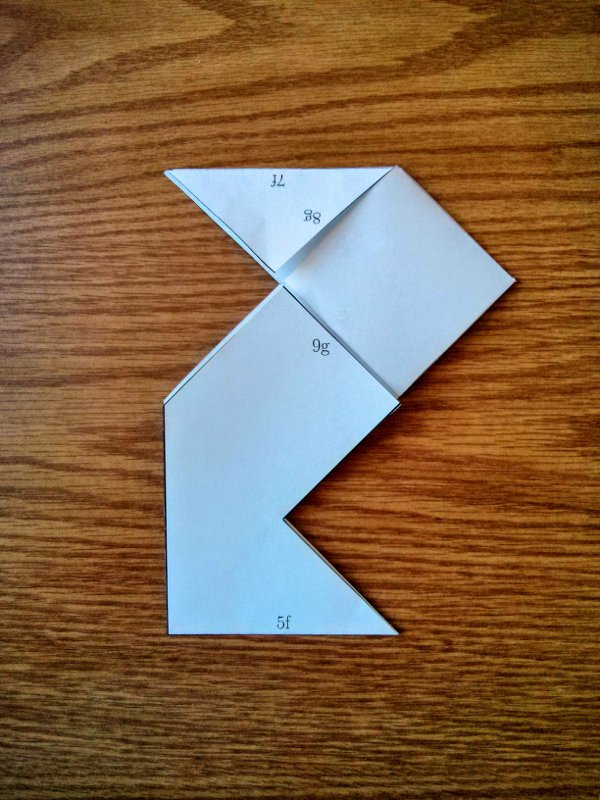5. The final view